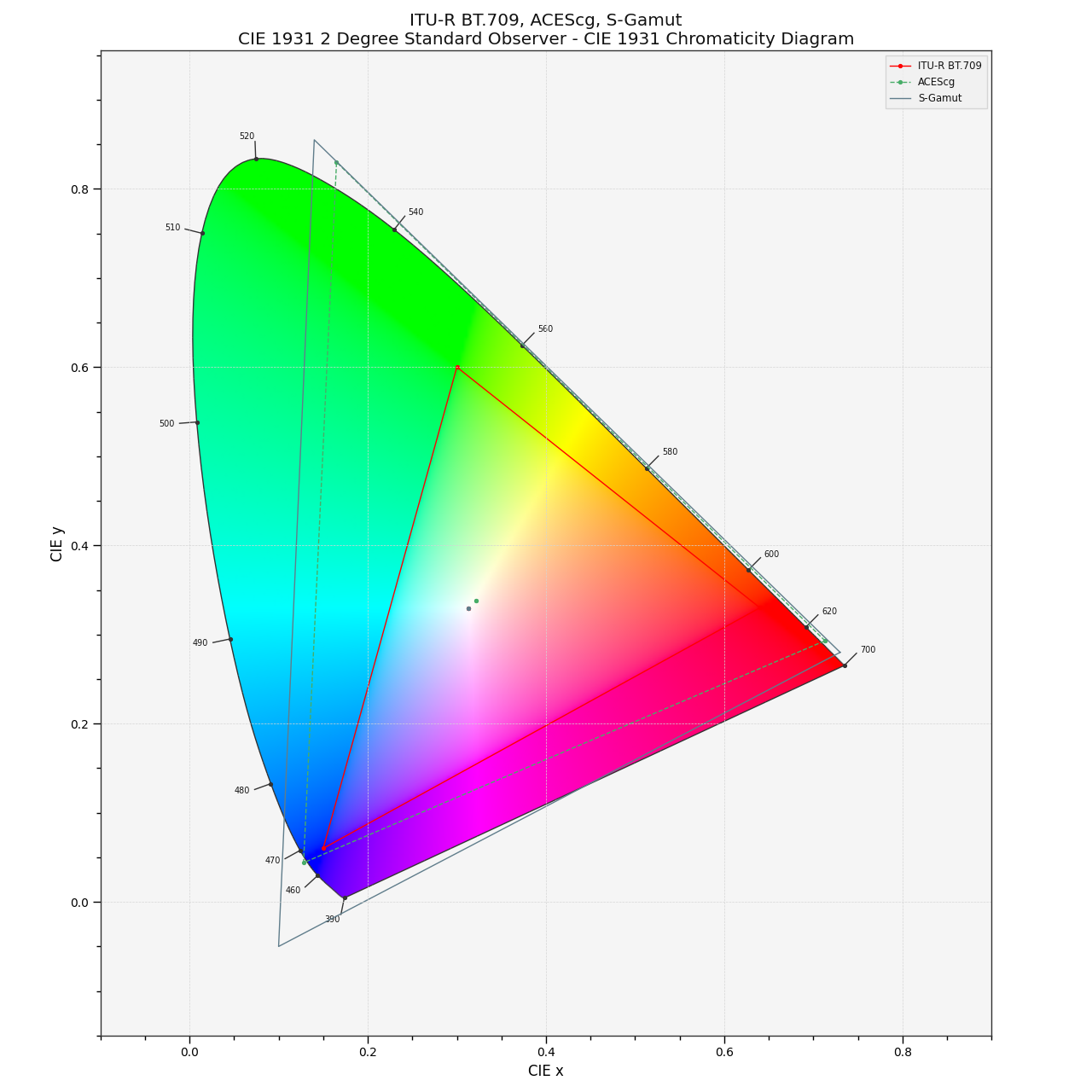colour.plotting.models.plot_RGB_colourspaces_in_chromaticity_diagram¶

colour.plotting.models.plot_RGB_colourspaces_in_chromaticity_diagram(colourspaces=None, cmfs='CIE 1931 2 Degree Standard Observer', chromaticity_diagram_callable=<function plot_chromaticity_diagram>, method='CIE 1931', show_whitepoints=True, show_pointer_gamut=False, **kwargs)[source]

Plots given RGB colourspaces in the Chromaticity Diagram according to given method.

Parameters: Other Parameters: colourspaces (array_like, optional) – RGB colourspaces to plot. cmfs (unicode, optional) – Standard observer colour matching functions used for Chromaticity Diagram bounds. chromaticity_diagram_callable (callable, optional) – Callable responsible for drawing the Chromaticity Diagram. method (unicode, optional) – {‘CIE 1931’, ‘CIE 1960 UCS’, ‘CIE 1976 UCS’}, Chromaticity Diagram method. show_whitepoints (bool, optional) – Whether to display the RGB colourspaces whitepoints. show_pointer_gamut (bool, optional) – Whether to display the Pointer’s Gamut. **kwargs (dict, optional) – {colour.plotting.artist(), colour.plotting.diagrams.plot_chromaticity_diagram(), colour.plotting.plot_pointer_gamut(), colour.plotting.render()}, Please refer to the documentation of the previously listed definitions. Current figure and axes. tuple

Examples

>>> plot_RGB_colourspaces_in_chromaticity_diagram(
...     ['ITU-R BT.709', 'ACEScg', 'S-Gamut'])
... # doctest: +SKIP